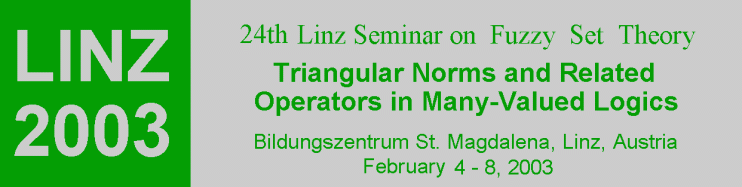Home

Since their inception in 1979 the Linz Seminars on Fuzzy Sets have emphasized the development of mathematical aspects of fuzzy sets by bringing together researchers in fuzzy sets and established mathematicians whose work outside the fuzzy setting can provide direction for further research. The seminar is deliberately kept small and intimate so that informal critical discussion remains central. There are no parallel sessions and during the week there are several round tables to discuss open problems and promising directions for further work. LINZ 2003 will be already the 24th seminar carrying on this tradition.

LINZ 2003 will deal with the use of Triangular Norms and Related Operators in Many-Valued Logics and their applications. Though the basic results in the theory of t-norms go back to the sixties, there is an important growth of interest in the theoretical background of t-norms and related operators (such as copulas, implications, uninorms, etc.) during the last years. Theory and applications of t-norms and related operators influence each other, as can be seen not only in probabilistic metric spaces, but also in many-valued logics, measure and integration theory, preference modelling, etc. For practical purposes, the determination of an appropriate t-norm fitting the observed data becomes an acute problem. The aim of the Seminar is an intermediate and interactive exchange of recent results. We expect that the presented talks will provide a comprehensive mathematical framework for the theory and application of triangular norms and related operators.

The Seminar will consider the following aspects without being strictly limited to them:

• Structure of t-norms
• Analytical and algebraic properties of t-norms
• Construction methods of t-norms
• Approximation of t-norms
• Related operators (t-conorms, copulas, implications, uninorms, etc.)
• Functional equations
• Probabilistic metric spaces
• Many-valued logics
• Preference modelling